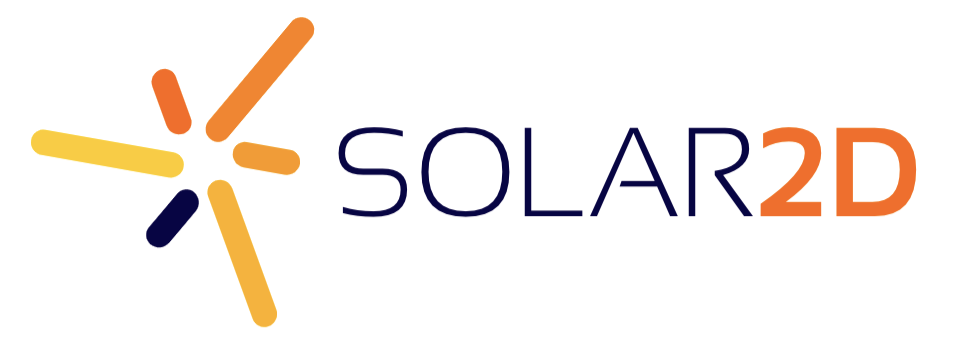# Draw curved lines ?

How can i draw curved lines in corona sdk??

1 Like

There are a couple of bezier curve projects in the Community code that you can search for.  There isn’t a native “curve” API.

There are a couple of bezier curve projects in the Community code that you can search for.  There isn’t a native “curve” API.

Is there any simple tutorial for drawing a curve?

I like cubic curve``````local bezierCubicCurve = function (t, p1, tg1, tg2, p2)
local x = (1 - t) * (1 - t) * (1 - t) * p1.x + 3 * (1 - t) * (1 - t) * t * tg1.x + 3 * (1 - t) * t * t * tg2.x + t * t * t * p2.x
local y = (1 - t) * (1 - t) * (1 - t) * p1.y + 3 * (1 - t) * (1 - t) * t * tg1.y + 3 * (1 - t) * t * t * tg2.y + t * t * t * p2.y
return ({x = x, y = y})
end

local tPoint = {
{x = 20, y = 80},
{x = 180, y = 10},
{x = 280, y = 250},
{x = 110, y = 420}
}

for p = 1, #tPoint do
local circle = display.newCircle(tPoint[p].x, tPoint[p].y, 3)
end

local startPoint = tPoint
for t = 0, 100 do
local workPoint = bezierCubicCurve(t / 100, tPoint, tPoint, tPoint, tPoint)
local line = display.newLine(startPoint.x, startPoint.y, workPoint.x, workPoint.y)
line:setStrokeColor( 1, 0, 0, 1 )
line.strokeWidth = 1
startPoint = workPoint
end
``````